Roman Numerals
 To the Main Page    "Mathematische Basteleien"

What are Roman Numerals?
 ...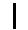... Roman numerals are the letters on the left, which are used for writing natural numbers.  On this page the (standard-) rules are described, which are (were?) taught in German schools.

Examples: LXIV=64, CCXXVI=226, CM=900, CMXCVIII=998 or MDCCXII=1712

You don't find the words Roman numbers in an encyclopedia or a reference book of mathematics. Otherwise you could think that the Romans had their own numbers and didn't use the natural numbers. Obviously Roman numbers mean the way of writing a number. - On the other hand you must not be too exact in number names. A number like MDCCXII is called a Roman number and is understood as that.
I say this because google.com  e.g. finds many web sites only with the string "roman numbers".

Values of Roman Numerals top
Each numeral has a certain value:
I=1      V=5      X=10      L=50      C=100      D=500       M=1000.

You can keep the values in your mind as follows.
>V is the upper half of X
>C stands for centum=100, known from centimeter=cm
>L is the lower half of C, if you have some imagination
>M stands for mille=1000, known from millimeter=mm
>D is the right half of (I), an old writing of 1000

You must not think that the numerals developed this way in former times.

Conversions   top
From the Roman to the Decimal System
First you look at numbers which are written in Roman numerals in decreasing values. There is always a numeral with a smaller value on the right side of a numeral. This is a number like MCCXII.
In this case you must only add the values: MCCXII = M+C+C+X+I+I = 1212.

Sometimes there are numbers with a numeral of a smaller value on the left side. This happens twice in CMXLVIII. Here you calculate the differences CM=M-C=1000-100=900 and XL=L-X=50-10=40 first and then you add:
CMXLVIII = CM+XL+V+I+I+I = 900+40+5+1+1+1=948.

From the Decimal to the Roman System
If you have the opposite problem to convert a number written in Arabic numerals to one in Roman numbers, choose numbers without 4 or 9 in the beginning.
An example is 1687. You break the number into thousands, hundreds, tens and units. In a second step you also consider the numbers 5, 50 and 500 (if possible) in the reduction.
1687=1000+600+80+7=1000+500+100+50+10+10+10+5+1+1=MDCLXXXVII
In the end you use Roman numerals.

If the number has 4 or 9, you must take differences.
Take the example 1942. Use the difference M-C=CM for 900 and L-X=XL for 40. Then you add:
1942 = 1000+900+40+2 = M+CM+XL+II = MCMXLII.

Maybe this way of using differences is confusing. Obviously one wanted to avoid four equal letters side by side.
IIII=IV=4    VIIII=IX=9    XXXX=XL=40     LXXXX=XC=90    CCCC=CD=400     DCCCC=CM=900.
Only these six differences are allowed. You can understand other figures like VM=995 or IC=99 easily and they are elegant, but if you allow them, there are different writings of the same number. So 995=VM=CMXCV and 99=IC=XCIX would be possible.

And where is the sign for the Roman zero? There is none, because there is no need for zero.

To the History of Roman Numerals    top
If you want to describe the history of these numerals, you must go far afield. I will restrict myself to three remarks.

The "old Romans" used four equal numerals like IIII, XXXX, CCCC and MMMM and didn't allow differences.

The letters D and M as numerals  came up later.

The Roman numerals spread in many countries up to the end of the Middle Ages. So you can understand that there were and are  many different ways of writing the numbers during the centuries. In this respect the rules I describe on this page and which are used in schools are constructed in some way, but have become standard today. Maybe the main reason is that the writing is definite

Chronograms   top
When letters are used as numerals, there are ingenious connections between words/sentences and numerals.
There is the number M+M+I+C+L+I=2152 in MATHEMATISCHE BASTELEIEN. (This isn't ingenious, but an example.)
The equation EINTAUSENDUNDZWEI=1002 is well known.

If the sum is a date, you call words or sentences chronograms. The Priest Johann Loofher lived in my hometown Bad Salzuflen around 1630. He designed Latin house inscriptions as chronograms for several houses. The year of building was hidden in the inscriptions. You can read more about this on my page chronograms

666     top
The sum of six of the seven numerals is D+C+L+X+V+I=666. The letter M is missing. This is a nice playing with numbers and results from the pairs (1,5), (10,50) und (100,500).
It is more interesting that 666 is the largest triangular number of equal numerals. There is 666=1+2+3+...+35+36=36*37/2.

The number 666 came in twilight, because it was called the "number of the animal" in the Bible:
Hier ist Weisheit! Wer Verstand hat, der überlege die Zahl des Tieres; denn es ist eines Menschen Zahl, und seine Zahl ist sechshundertsechsundsechzig (Offenbarung des Johannes 13,18 in Luthers Übersetzung).
In interpretations of the Bible the number of the animal is a bad number and is also called Number of the Beast, Satan's Number or Antichrist's Number.

Consequently people found 666 in the Roman emperors' names Nero and Deocletian, because they persecuted Christians.
In the 16th century, the times of the Religious Wars, 666 was connected with Luther's name and - in reverse - with that of the Pope.
(Book 2, Seite 347 ff.)

The Pope's example uses the principle of the chronogram.
The Pope was called VICARIUS FILII DEI (Representative of God's son). If you add the values of the Roman numerals, you get 666 (VICARIVS FILII DEI).

Look on the internet with the string 666 and you are flooded with informations, if you like.

Playings with Matches top
There is a tradition to lay equations with matches, which are obviously wrong. If you only move one match, the equation becomes correct.
Here are some classics among others.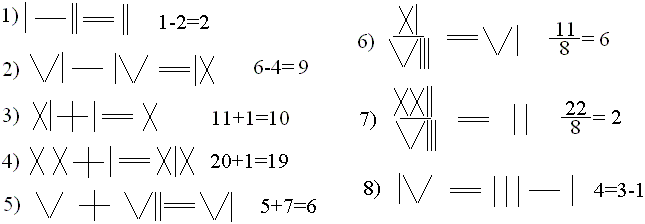The solutions are at the bottom of this page. - It is easy to discover more examples.

Roman Numerals Today top
Today you don't use Roman numerals often. You use them for numbering chapters in books or pages of forewords or list of contents depending on taste or fashion.
Sometimes dates are given in Roman numerals, for instance for houses or in connection with the copyright of books.
Even Words for Windows is able to number lines automatically  [ :-(  ]  in Roman numerals.

near
Most frequently you see Roman numerals on clocks.

Watch maker shops use large clocks for gaining attention. They prefer decorative Roman faces to show their high quality.Clock in the Begastraße of  Schötmar, part of Bad Salzuflen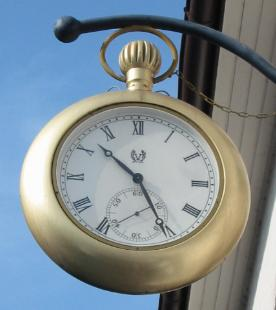A second clock hangs in the Brüderstraße
It is conspicuous that most of the Roman faces have letters directed to the centre and that IV is written as IIII. So there is no confusion with VI (=I/\).
This is one explanation. Please look at Gordon T. Uber's page, URL below for further informations.One of three clocks of the Stadtkirche (town church) follows.Clock at the former high school and Volkshochschule (adult education centre) now.Clock in the gable of a farm house in Rhiene.  The clock was at the tower of the village church of Lieme.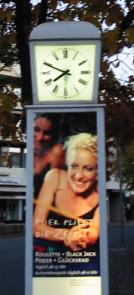Clock in the Bismarckstraße.  It is at the top of an advertisement tower for the casino  at Bad Oeynhausen.

far away
 ............. You can visit a strange clock in the nice village Siána on the island of Rhodos in Greece.  There are two clock towers beside the village church. One has a clock and it always tells the time 9.33 o'clock.
It is only painted ;-). Today the village could afford a real clock because of the many tourists who visit the village. But why should it loose such an attraction?

Roman Numerals on the Internet    top

German

Horst Hicke (Unterrrichtsmaterial-schule.de)
Römische Zahlen

matroid
Römische Ziffern

Numeralia - Zahlwörter

Wolfgang Back
Römische Zahlen

English

Christopher Handy
Roman Numeral Year Dates

Gordon T. Uber
FAQ: Roman IIII vs. IV on Clock Dials

Jordan Allan
Roman Numerals

novaroma
On Roman Numerals

Paul Lewis
ROMAN NUMERALS AND CALENDAR

Wikipedia
Roman numerals, Number of the Beast

Solutions   top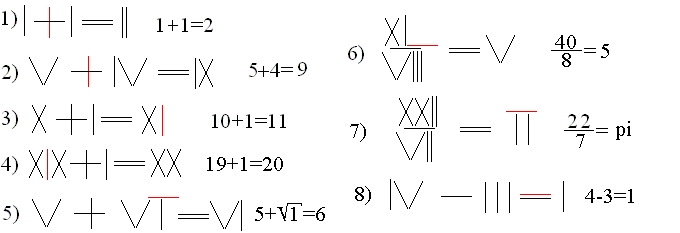References   top
(1) Jan Gullberg: Mathematics - From the Birth of Numbers, New York / London 1997 [ISBN 0-393-04002-X]
(2) Georges Ifrah: Universalgeschichte der Zahlen, Köln 1998 [ISBN 3-88059-956-4]
(3) Johannes Lehmann: So rechneten Griechen und Römer, Reinhardt Becker Verlag [ISBN 3-930640-11-2]
or (3) Johannes Lehmann: So rechneten Griechen und Römer, Urania , Leipzig, Jena, Berlin , 1994 [ISBN 3-332-00522-7]

Thank you Gail from Oregon Coast for supporting me in my translation.

Feedback: Email address on my main page